GMAT  >  Test: Unit Digit- 2

# Test: Unit Digit- 2 - GMAT

Test Description

## 10 Questions MCQ Test Quantitative for GMAT - Test: Unit Digit- 2

Test: Unit Digit- 2 for GMAT 2023 is part of Quantitative for GMAT preparation. The Test: Unit Digit- 2 questions and answers have been prepared according to the GMAT exam syllabus.The Test: Unit Digit- 2 MCQs are made for GMAT 2023 Exam. Find important definitions, questions, notes, meanings, examples, exercises, MCQs and online tests for Test: Unit Digit- 2 below.
Solutions of Test: Unit Digit- 2 questions in English are available as part of our Quantitative for GMAT for GMAT & Test: Unit Digit- 2 solutions in Hindi for Quantitative for GMAT course. Download more important topics, notes, lectures and mock test series for GMAT Exam by signing up for free. Attempt Test: Unit Digit- 2 | 10 questions in 20 minutes | Mock test for GMAT preparation | Free important questions MCQ to study Quantitative for GMAT for GMAT Exam | Download free PDF with solutions
 1 Crore+ students have signed up on EduRev. Have you?
Test: Unit Digit- 2 - Question 1

### Find the unit digit of (432)412 × (499)431

Detailed Solution for Test: Unit Digit- 2 - Question 1

Given:
(432)412 × (499)431
Concept:
9even no. = unit digit 1
9odd no. = unit digit 9
Calculation:
(432)412 × (499)431
Taking unit digits
⇒ 2412 × 9431
As we know unit digit of
21 = 2, 22 = 4, 23 = 8, 24 = 6
⇒ 24(103) × 9431
⇒ 6 × 9
⇒ 54
∴ The unit digit of (432)412 × (499)431 is 4.

Test: Unit Digit- 2 - Question 2

### What is the units digit of (a +b)2 – (a-b)2, where a and b are non-negative integers?        (1) The difference between any two consecutive multiples of a is 5        (2) b when multiplied by any even integer results in the same units digit, not necessarily equal to units digit of b.

Detailed Solution for Test: Unit Digit- 2 - Question 2

Steps 1 & 2: Understand Question and Draw Inferences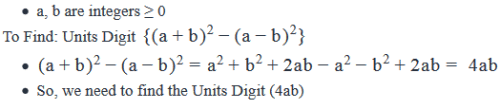Step 3: Analyze Statement 1 independently

1. The difference between any two consecutive multiples of a is 5

• Let the consecutive multiples of a be a*n and a*(n+1)
• So, a(n+1) – an = 5
• an + a – an = 5
• a = 5

If a = 5, 4ab = 20b. So, irrespective of the value of b, the units digit(20b) = 0.

Step 4: Analyze Statement 2 independently

2.  b when multiplied by any even integer results in the same units digit, not necessarily equal to units digit of b.

• Following cases are possible:

• Units Digit(b) = 0.
• 0 when multiplied with any integer will have the Units Digit as 0. In this case the units digit(4ab) = 0.

• Units Digit(b) = 5.
• 5 when multiplied with any even integer will result in units digit of 0. So, Units Digit(4ab) = 0.

As we have a unique answer of Units Digit(4ab), this statement is sufficient to answer.

Step 5: Analyze Both Statements Together (if needed)

As we have a unique answer from steps 3 and 4, this step is not required.

Test: Unit Digit- 2 - Question 3

### What is the units digit of zx, where x and z are positive integers?      (1)   z when divided by 100 has its hundredths digit as 5      (2)  The product of z2 and z3 has the same units digit as z2.

Detailed Solution for Test: Unit Digit- 2 - Question 3

Steps 1 & 2: Understand Question and Draw Inferences

• x, z are integers > 0

To Find: Units Digit (zx)

Step 3: Analyze Statement 1 independently

1. z when divided by 100 has its hundredths digit as 5

• Let z = abcdef, where f is the units digit.
• So, z100=abcd.ef

The hundredths digit of z100

•  = f = Units Digit (z) = 5

Now, a number with its Units Digit = 5, raised to any power will always have its Units Digit as 5.

So, Units Digit (zx) = 5

(Note: Even if you took z to have, say, 8 digits – a number like z = abcdefgh, where a to h are digits – you would still get the units digit of z (the units digit is h in this case) as 5. Therefore, the answer would still be the same)

Step 4: Analyze Statement 2 independently

2. The product of z2 and z3 has the same units digit as z2.

• Product of z2 and z3 = (z2)(z3) = z2+3 = z5
• Units Digit (z5) = Units Digit (z2). This equation will be satisfied in the following cases:
• Case 1: If the units digit of z2 has a cyclicity of 3
• However, we know that none of the digits have a cyclicity of 3
• Therefore, this case is rejected
• Case 2: If the units digit of z2 has a cyclicity of 1
• The digits that satisfy this case are: { 0, 1, 5, 6}
• So, Units Digit(z) = { 0, 1, 5, 6}

As we do not have a unique value of Units Digit of(z), this statement is insufficient to answer.

Step 5: Analyze Both Statements Together (if needed)

As we have a unique answer from step-3, this step is not required.

Test: Unit Digit- 2 - Question 4

For any positive number n, the function #n represents the value of the number n rounded to the nearest integer. If k is a positive number, what is the units digit of #k?

(1)  #(10k) = 10k

(2)  #(100k) is 10300.

Detailed Solution for Test: Unit Digit- 2 - Question 4

Steps 1 & 2: Understand Question and Draw Inferences

Given:

• Let k = n + a.bcde. . . , where n is a multiple of 10 and a, b, c, d, e etc. denote the units, tenths, hundredths, thousandths, ten-thousandths digits of k respectively.

• Example: Say k is equal to 123.789. We can also write this value as 120 + 3.789. This form is comparable to n + a.bcde . . .

• So, n denotes the value of all the digits at the tens, hundreds, thousands and higher place values (if k = 123.789, n = 120; if k = 3456.987, n = 3450; if k = 43789.34, n = 43780 and so on)

• We have split k into n and a.bcde. . . because the question doesn’t indicate whether the magnitude of k is in tens or hundreds or thousands etc. (that is, is k a number like 12.345 or like 123.789 or like 3456.987 etc.) This knowledge is not important either because all the action is happening at the units digit and the digits to the right of the decimal point. So, we’ve simply expressed the value of all the digits at the tens, hundreds, thousands and higher place values in a single term n

• #k =
• n + a, if b < 5
• n + (a+1) if b ≥ 5

To find:

• The units digit of #k
• Since n is a multiple of 10, from the expression of #k, we can say that the units digit of #k =
• a, if b < 5
• a + 1, if b ≥ 5

Thus, to answer the question, we need to know the value of a and whether b < 5 or not

Step 3: Analyze Statement 1 independently

(1)  #(10k) = 10k

• 10k = 10n + ab.cde. . .
• Since a is now the tens digit and b is the units digit, we can write

10k = 10n + 10a + b.cde. . .

10k = 10(n+a) + b.cde. . .

• Applying the definition of the function #n, we can write:
• #(10k) =
• 10(n+a) + b if c < 5
• 10(n+a) + (b+1) if c ≥ 5
• We are given that #(10k) = 10k.
• #(10k) = 10(n+a) + b.cde . . .

• If c < 5, then by substituting the value of #(10k) in the above equation, we get:
• 10(n+a) + b = 10(n+a) + b.cde. . .
• b = b + 0.cde. . .
• This implies, c = d = e . . .= 0
• Thus, k is a number of the form n + a.b, where b is the sole digit after the decimal point

• If  c ≥ 5, then by substituting the value of #(10k) in the above equation, we get:
• 10(n+a) + (b+1) = 10(n+a) + b.cde. . .
• b  +1 = b + 0.cde . . .
• 1 = 0.cde . .  .
• For no values of digits c, d, e . . . will the above equation be satisfied.
• Therefore, this case is not possible

• Thus, from Statement 1, we conclude that k is a number of the form n + a.b, where b is the sole digit after the decimal point
• However, we still don’t know the value of a or whether b is less than 5 or not.

So, Statement 1 alone is not sufficient.

Step 4: Analyze Statement 2 independently

(2) #(100k) is 10300.

• 100k = 100n + abc.de. . .
• 100k = (100n + 100a + 10b) + c.de. . .

• Applying the definition of the function #n, we can write:
• #(100k) =
• (100n + 100a + 10b) + c if d < 5
• (100n + 100a + 10b) + (c+1) if d ≥ 5

• We are given that #(100k) = 10300
• If d < 5, then we can write
• (100n + 100a + 10b) + c = 10300
• Remember that in the above expression, a, b and c are digits (therefore, lie between 0 and 9, inclusive) whereas n is a multiple of 10.
• Comparing the units digits on both sides of the equation, we get: c = 0
• Comparing the tens digits on both sides of the equation, we get: b = 0
• Comparing the hundreds digits on both sides of the equation, we get: a = 3
• Since b < 5 in this case, the units digit of #k = a = 3

• If d ≥ 5, then we can write
• (100n + 100a + 10b) + (c+1) = 10300
• Comparing the units digits on both sides of the equation, we get: c + 1 = 0
• So, c = 9 and 1 is carried over to tens place
• Comparing the tens digits on both sides of the equation, we get: b + 1 = 0 (Note, we’re writing b+1 and not b due to the carry-over)
• So, b = 9 and 1 is carried over to hundreds place
• Comparing the hundreds digits on both sides of the equation, we get: a + 1 = 3
• So, a = 2
• Since b > 5 in this case, the units digit of #k = a  +1 = 3

• Thus, we see that both possible values of #(100k) lead to the same value of the units digit of #k
• Thus, Statement 2 is sufficient to find a unique answer to the question.

Step 5: Analyze Both Statements Together (if needed)

Since we’ve already arrived at a unique answer in Step 4, this step is not required

Test: Unit Digit- 2 - Question 5

Arrange the following terms in the increasing order of their units digits:

I. 75

II. 86

III. 123

Detailed Solution for Test: Unit Digit- 2 - Question 5

Given: 3 terms: 75, 86 and 123

To find: The correct increasing order of the units digits of the 3 terms

Approach:

1. We will first find the units digit of each term by using the cyclicity of units digits
2. Then, we will arrange the units digits in increasing order.

Working Out:

• Finding the units digit of Term I - 75
• We know that 7 has a cyclicity of 4
• Now, 5 = 4 + 1
• So, the units digit of 75 will be the same as the units digit of 71 = 7
• So, units digit of Term I is 7

• Finding the units digit of Term II - 86
• We know that 8 has a cyclicity of 4
• Now, 6 = 4 + 2
• So, the units digit of 86 will be the same as the units digit of 82 = 64
• So, units digit of Term II is 4

• Finding the units digit of Term III - 123
• The units digit of 123 will come from 23 = 8
• So, units digit of Term III is 8

• Arranging the units digits in increasing order, we get: 4 < 7 < 8
• That is, II < I < III
• Looking at the answer choices, we see that the correct answer is Option D
Test: Unit Digit- 2 - Question 6

For any positive number x, the function [x] denotes the greatest integer less than or equal to x. For example,  = 1, [1.367] = 1 and [1.999] = 1.

If k is a positive integer such that k2 is divisible by 45 and 80, what is the units digit of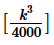Detailed Solution for Test: Unit Digit- 2 - Question 6

Given:

• Positive integer k (so, k > 0)
• k2 is divisible by 45
• k2 is divisible by 80
• The function [x]

To find: The units digit ofApproach:

1. To find the units digit of [x], we need to know or at least have some idea of the value of x. So, in order to answer the question, we need to find the value or at least a clue about the value of the number1. This number contains k3, which is the cube of a positive integer. However, the given information is about k2. Like in all questions that involve divisibility information about different powers of an integer, we’ll work out information about k3 as under:
• First use the information given about k2 to infer about the prime-factorized form of k.
• Then, by cubing the prime-factorized expression of k, get an expression for k3

Working Out:

• Inferring the prime-factorized expression for k from the given information
• Let k = P1a * P2b *P3c *P4d  . . .where P1, P2 etc. are prime numbers and a, b . . . are non-negative integers
• Therefore, k2 = P12a * P22b *P32c *P42d  . . .
• We are given that k2 is divisible by 45
• 45 = 32*5
• This means, P1 = 3 and 2a ≥ 2
• That is, a ≥ 1
• And, P2 = 5 and 2b ≥ 1
• That is,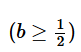• Since b is an integer, minimum possible value of b = 1
• Therefore, k = (31*51)(3x*5y* P3c *P4d  . . .) where x and y are non-negative integers
• Note: In the above expression, we’ve taken 31 and 51 outside the remaining expression in order to emphasize the fact that k definitely does contain 31 and 51, whether or not it contains higher powers of 3 and 5
• So far, we’ve inferred that: k = (31*51)(3x*5y* P3c *P4d  . . .)
• So, k2 = (32*52)(32x*52y* P32c *P42d  . . .)
• We are also given that k2 is divisible by 80
• 80 = 24*5
• This means, P3 = 2 and 2c ≥ 4
• That is, c ≥ 2
• So, k = (22*31*51)(2z*3x*5y* P4d  . . .), where x, y, z, d etc. are non-negative integers
• Inferring the expression for k3
• Using the expression for k inferred above, we can write:
• k3 = (26*33*53)(23z*33x*53y* P43d  . . .),
• Drawing inferences about the value of• 4000 = 25*53
• Looking at the expression for k3 above, we see that k3 will be divisible by 4000

• So,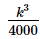is an integer
• The value of this integer will be (21*33)(23z*33x*53y* P43d  . . .)
• So,= (21*33)(23z*33x*53y* P43d  . . .)
• If y ≥ 1, then the units digit of the right hand side expression will be 0 (because the units digit of 2*5 is 0. Note that the above expression has at least one 2)
• But if y = 0, then the units digit will depend on the power of 2, 3 and the value and powers of any other prime numbers that are present in k
•

• Thus, we are not able to determine a unique value of the units digit of• Looking at the answer choices, we see that the correct answer is Option E

Test: Unit Digit- 2 - Question 7

n is a positive integer that lies between 100 and 200, exclusive, and has no digits repeated. Is the units digit of n equal to 5?

(1)  n is divisible by 9 and the 2-digit number formed by inverting the units and tens digit of n is a prime number.

(2)  The difference between the units digit and the tens digit of n is the same as the difference between the tens digit and the hundreds digit of n and is equal to 2.

Detailed Solution for Test: Unit Digit- 2 - Question 7

Steps 1 & 2: Understand Question and Draw Inferences

• n is an integer with no repeated digits such that 100 < n < 200
• From the above inequality, it is clear that Hundreds digit(n) = 1
• Let the number n = 1bc, where b and c are the tens and units digits of n respectively.

To Find: Is Units Digit(n) = 5?

• That is, is c = 5?

Step 3: Analyze Statement 1 independently

1. n is divisible by 9 and the 2-digit number formed by inverting the units and tens digit of n is a prime number.
• The number cb is a prime number
• Also, since n is divisible by 9, the sum of the digits of n must be divisible by 9
• Therefore, 1 + b + c must be divisible by 9
• So, 1 + b + c = { 9, 18}.
• It cannot be equal to 27 or more as the maximum possible sum of b + c = 9 +8 = 17 (Remember that the digits of n are distinct. So, if one digit is 9, the maximum value of the other digit can be 8).
• So, following cases are possible:
• Either b + c = 8. Possible combinations of two distinct positive integers adding up to 8 can be:

• (b, c) = (2, 6). In this case cb = 62, which is not a prime number. So, this case is not possible.
• (b, c) = (3, 5). In this case, cb = 53, which is a prime number. So, this is a possible case.
• (b, c) = (5, 3). In this case cb = 35, which is not a prime number. So, this case is not possible
• (b, c) = (6, 2). In this case cb = 26, which is not a prime number. So, this case is not possible.

• Or b + c = 17. In this case, (b, c) = (8, 9) in any order. Following cases are possible:

• If (b, c) = (8, 9), then cb = 98, which is not a prime number. So, this case is not possible
• If (b, c) = (9, 8), then cb = 89, which is a prime number. So, this is a possible case.

Hence, the possible values of n = { 135, 198}. So, the units digit of n = {5, 8}

As we do not have a unique value of units digit(n), the statement is insufficient to answer.

Step 4: Analyze Statement 2 independently

2.   The difference between the units digit and the tens digit of n is the same as the difference between the tens digit and the hundreds digit of n and is equal to 2.

• We’ve already inferred in Steps 1 and 2 that Hundreds digit(n) = 1
• So, Tens Digit(n) = 1 + 2 = 3
• And, Units Digit(n) = 3 + 2 = 5

Thus, the units digit(n) = 5

As we have a unique value of units digit(n) , this statement is sufficient to answer.

Step 5: Analyze Both Statements Together (if needed)

As we have a unique value from step 4, this step is not required.

Test: Unit Digit- 2 - Question 8

If p and q are positive integers, what is the remainder when (27)12p + (3)6q is divided by 5?

(1) p is an odd prime number

(2) q is divisible by 10

Detailed Solution for Test: Unit Digit- 2 - Question 8

Steps 1 & 2: Understand Question and Draw Inferences

Given: Positive integers p and q

To find:

• The remainder when (27)12p + (3)6q is divided by 5
• The remainder when an expression is divided by 5 is determined by the units digit of the expression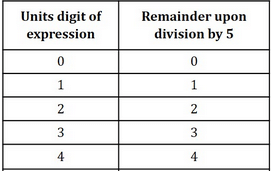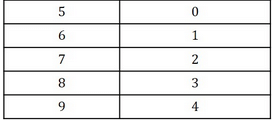• The units digit of the expression is obtained from the sum of the units digits of (27)12p and (3)6q
1. Units digit of 2712p = Units digit of 712p = Units digit of (74)3p = Units digit of 13p = 1
2. Units digit of (3)6q = Units digit of (32)3q = Units digit of (9)3q
• If q is even, units digit of (9)3q  = units digit of 9even = 1
• If q is odd, units digit of (9)3q = units digit of 9odd = 9

• Thus, the units digit of the expression is equal to
1. 1 + 1 = 2 (if q is even)
2. 1 + 9 = 0 (if q is odd)

• Correspondingly, the remainder when the expression is divided by 5 is equal to
1. 2 (if q is even)
2. 0 (if q is odd)

So, to answer the question, we need to know if q is even or odd.

Step 3: Analyze Statement 1 independently

(1) p is an odd prime number

Statement 1 gives no information about q’s even-odd nature.

So it’s not sufficient to answer the question

Step 4: Analyze Statement 2 independently

(2) q is divisible by 10?

• So, q is of the form 10k, where k is a positive integer
• Thus, q is even

Since we now know the even-odd nature of q, we can answer the question. Statement 2 alone is sufficient.

Step 5: Analyze Both Statements Together (if needed)

Since we’ve already arrived at a unique answer in Step 4, this step is not required

Test: Unit Digit- 2 - Question 9

If x and y are distinct positive integers and x+y is even, what is the remainder when (x+y)a is divided by 10, where a is a positive integer?

(1) Units digit of y is 6

(2) (xy)a is divisible by 10.

Detailed Solution for Test: Unit Digit- 2 - Question 9

Steps 1 & 2: Understand Question and Draw Inferences

• x, y are distinct integers > 0 such that x + y = even
• This means, Either both x andy  are even
• Or both x andy are odd
• a is an integer > 0

To Find: the value of r in (x+y)a=10k+r, where k is the quotient obtained when (x+y)a  is divided by 10 and r is the remainder; so,  0 ≤ r < 10

• Now, when a number is divided by 10, the remainder  is equal to the units digit of that number.
• So, r= units digit of (x+y)a

Step 3: Analyze Statement 1 independently

1. Units digit of y is 6

• It does not tell us anything about the units digit of x as well as about the value of a.

Step 4: Analyze Statement 2 independently

2.   (xy)a is divisible by 10.

• As (xy)a is divisible by 10, the units digit of (xy)a = 0
• So, the units digit of xy = 0. Two cases are possible:
• Units digit of (x, y) = { 5, even number) in any order. However in this case the number with 5 as its units digit will be odd and the other number will be even. However, we’ve deduced in Steps 1 and 2 that x and y have the same even-odd nature. So, this case is not possible as it contradicts the given information (that the sum x + y is even).
• Units digit of (x, y) = (0, even number) in any order. In this case x and y are both even. So, this case is possible.
• However since we do not have a unique value of units digit of both x and y and we do not know the value of a, we cannot find a unique value of the units digit of (x+y)a

Step 5: Analyze Both Statements Together (if needed)

1. From Statement 1, we know that Units digit of y = 6
2. From Statement 2, we inferred that Units digit of (x, y) = (0, even number) in any order

Combining both the statements, we can say that units digit (x) = 0 and units digit(y) = 6

So, units digit of (x+y) = 6. Now do we need the value of a to find out the units digit of (x+y)?

We know a number with units digit of 6 raised to any power always results in units digit of 6.

So, Units Digit ((6a)) = 6.

Thus r = Units Digit ((6a)) = 6.

Test: Unit Digit- 2 - Question 10

If a and b are positive integers, is the sum a + b divisible by 4?

1. When the sum 23a + 25b is divided by 10, the remainder is 8
2. When 22b is divided by 10, the remainder is 8
Detailed Solution for Test: Unit Digit- 2 - Question 10

Steps 1 & 2: Understand Question and Draw Inferences

Given: Integers a, b > 0

To find: If a + b is divisible by 4?

Step 3: Analyze Statement 1 independently

Statement 1 says that ‘When the sum 23a + 25b is divided by 10, the remainder is 8’

• Now, we know that when an integer is divided by 10, the remainder is equal to the units digit of that integer
• For example, when 25 is divided by 10, the remainder is 5 (the units digit of 25)
• So, from Statement 1, we can infer that the units digit of the sum 23a + 25b is 8
• Now, the units digit of 25b will always be 5, for all possible values of the positive integer b
• Therefore, the units digit of the term 23a must be 3
• Now, the units digit of 23a comes from 3a
• For the units digit to be 3, a must be of the form 4k + 1, where k is a non-negative integer
• Thus, from Statement 1, we have inferred that a is of the form 4k + 1. However, since we do not yet know about the value of b, we cannot comment if the sum a + b is divisible by 4 or not.
• So, Statement 1 is not sufficient.

Step 4: Analyze Statement 2 independently

Statement 2 says that ‘When 22b is divided by 10, the remainder is 8’

• We know that when an integer is divided by 10, the remainder is equal to the units digit of that integer
• So, from Statement II, we can infer that the units digit of 22b is 8
• Now, the units digit of 22b comes from 2b
• For the units digit to be 8, b must be of the form 4m + 3, where m is a non-negative integer

• Thus, from Statement 2, we have inferred that b is of the form 4m + 3. However, since we do not know about the value of a, we cannot comment if the sum a + b is divisible by 4 or not.
• So, Statement 2 is not sufficient.

Step 5: Analyze Both Statements Together (if needed)

• From Statement 1, we inferred that a is of the form 4k + 1
• From Statement 2, we inferred that b is of the form 4m + 3
• So, combining the 2 statements, we observe that the sum a + b is of the form 4k + 4m + 4
• Therefore the sum a + b is indeed divisible by 4
• Since we’ve arrived at a unique answer to the question, the two statements together are sufficient.(Scan QR code)